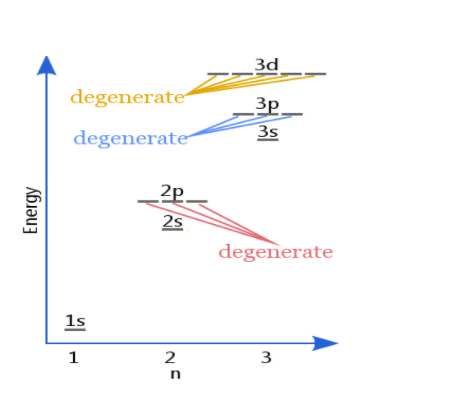# Degenerate Orbitals

## Degenerate orbitals definition:

Electron orbitals having the same energy levels are called degenerate orbitals.

As per the Aufbau principle, lower energy levels are filled before higher energy levels. As per Hund’s rule, degenerate orbitals are filled evenly before electrons are filled into higher energy levels. The Aufbau principle, Pauli’s exclusion principle, and Hund’s rule are the three rules that dictate the manner in which electrons are distributed into orbitals.

## What is the Aufbau Principle?

It states that in the ground state of an ion or an atom, the atomic orbitals of the electrons fill the lowest available energy levels before they occupy the higher levels. For instance, 2s subshell is filled after the 1s shell is occupied. Hence the most stable electron configuration is achieved.

## What is Hund’s rule?

Hund’s rule states that the greatest number of parallel electron spins happen in the ground state electron configuration in any electron subshell. This rule was formulated by Friedrich Hund in the year 1927. Hund’s rule can be used for determining the ground state of a molecule or an atom.

## Degenerate Orbitals Example:

p orbital has 3 degenerate orbitals. All three have the same energy levels. Each orbital is first assigned with only one electron. The second electron will be of opposite spin. Each orbital is filled and the total is six electrons.

## Explanation of Degenerate Orbitals with Diagram

Orbitals in the 2p sublevel are degenerate orbitals – Which means that the 2px, 2py, and 2pz orbitals have the exact same energy, as illustrated in the diagram provided below. Similarly, the 3px, 3py, and 3pz are degenerate orbitals. And at the 3d energy level, the 3dxy, 3dxz, 3dyz, 3dx2 – y2, and 3dz2 are degenerate orbitals with the same energy.Degeneracy – The total number of different states of the same energy is called degeneracy. It is also known as the degree of degeneracy.

• The degree degeneracy of p orbitals is 3
• The degree degeneracy of d orbitals is 5
• The degree degeneracy of f orbitals is 7